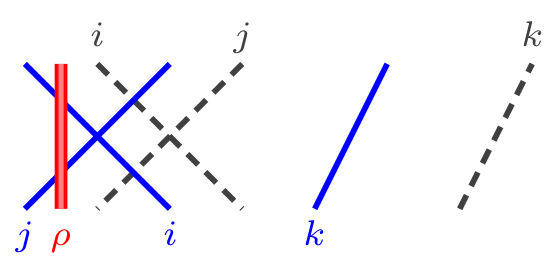## Abstract

Weighted KLRW algebras are diagram algebras generalizing KLR algebras. This paper undertakes a systematic study of these algebras culminating in the construction of homogeneous affine cellular bases in affine types $$A$$ and $$C$$, which immediately gives cellular bases for the cyclotomic quotients of these algebras. In addition, we construct subdivision homomorphisms that relate weighted KLRW algebras for different quivers. As an application we obtain new results about the (cyclotomic) KLR algebras of affine type, including (re)proving that the cyclotomic KLR algebras of type $$A^{(1)}_{e}$$ and $$C^{(1)}_{e}$$ are graded cellular algebras.

## A few extra words

Khovanov-Lauda and independently Rouquier introduced the so-called KLR algebras (or quiver Hecke algebras), motivated by questions in categorification, or more precisely from categorical actions of Lie algebras and the categorification of their simple highest weight modules. Webster further generalized these algebras to KLRW algebras, which give categorifications of tensor products of simple highest weight modules. All of these algebras admit finite dimensional quotients, called cyclotomic KLR(W) algebras. All of these algebras are graded algebras and all can be defined diagrammatically using generators and relations. Almost by definition, they play a crucial role in categorification and representation theory.
The discovery of these algebras and their properties started a major paradigm shift in representation theory. As important special cases these algebras include the group algebras of the symmetric group, its Hecke algebra, and the cyclotomic Hecke algebras of type A. The KLR algebras revealed deep new structures in their modules.
To define the weighted KLRW algebras Webster embellished the KLR algebras by introducing a weighting and additional strings to the diagrammatic presentation of the KLR algebras, and some subtle changes in the relations.
Here is an example of how the diagrams look like:These type of diagrams have three different strings: solid, ghost and red strings, all of which can be colored by vertices of the underlying quiver.
This paper undertakes a systematic study of these algebras.
The earlier sections of this paper apply with almost no restrictions on the choice of quiver but for the some results of the paper we restrict our attention to the quivers:In particular, in these types we construct homogeneous (affine) cellular bases for the weighted KLRW algebras and their finite dimensional quotients. The upshot of our construction is that the various cellular bases that we construct are essentially the same, regardless of the quiver (meaning those above) and whether we consider the infinite dimensional or the finite dimensional algebras. This makes use believe that the same construction will give rise to homogeneous (affine) cellular bases, or something close to such bases, for the following quivers: